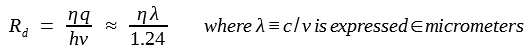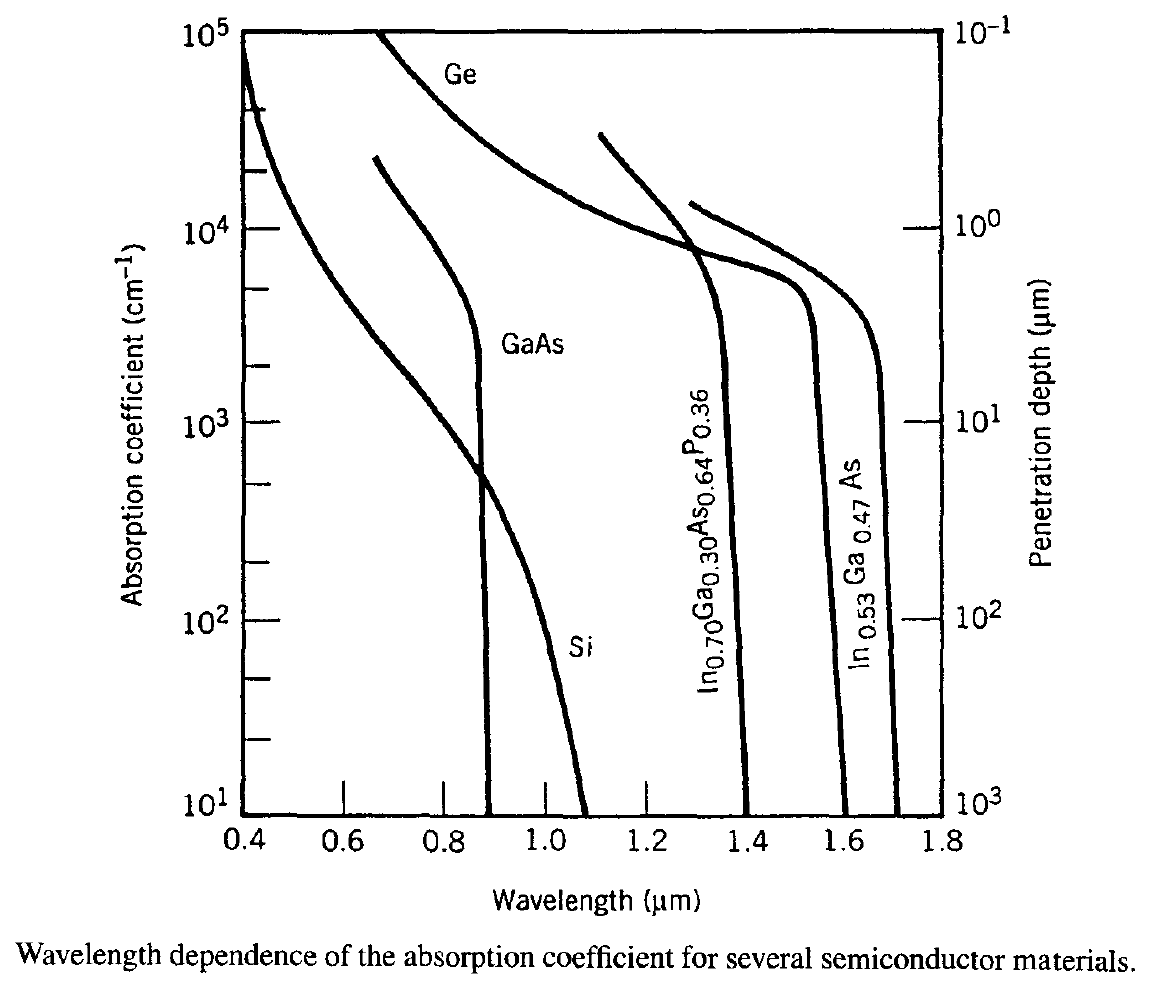# Basic Concepts of Optical Receivers

The role of an optical receiver is to convert the optical signal back into electrical form and recover the data transmitted through the lightwave system. Its main component is a photodetector that converts light into electricity through the photoelectric effect.The requirements for a photodetector are similar to those of an optical source. It should have high sensitivity, fast response, low noise, low cost, and high reliability. Its size should be compatible with the fiber-core size. These requirements are best met by photodetectors made of semiconductor materials.

The fundamental mechanism behind the photodetection process is optical absorption. This tutorial introduces basic concepts such as responsivity, quantum efficiency, rise time, and bandwidth that are common to all photodetectors and are used to characterize them.

#### 1. Resonsivity and Quantum Efficiency

Consider the semiconductor slab shown schematically in the following figure.If the energy of incident photons exceeds the bandgap energy, an electron-hole pair is generated each time a photon is absorbed by the semiconductor. Under the influence of an electric field set up by an applied voltage, electrons and holes are swept across the semiconductor, resulting in a flow of electric current. The photocurrent Ip is directly proportional to the incident optical power Pin, i.e.,

Ip = RdPin

The constant Rd is referred to as the responsivity of the photodetector because more current is produced at a given input power for larger values of Rd; it is expressed in units of ampere/watts (A/W).

The responsivity Rd can be expressed in terms of a fundamental quantity η, called the quantum efficiency and defined asThe responsivity Rd  is thus given byThe responsivity of a photodetector increases with the wavelength λ simply because more photons are present for the same optical power. Such a linear dependence on λ is not expected to continue forever because eventually the photon energy becomes too small to generate electrons. In semiconductors, this happens for < Eg, where Eg is the bandgap. The quantum efficiency η then drops to zero.

The dependence of η on λ enters through the absorption coefficient α. If the facets of the semiconductor slab in the figure above are assumed to have an antireflection coating, the power transmitted through the slab of width W is Ptr = exp(-αW)Pin. The absorbed power can be written as

Pabs = Pin - Ptr = [1 - exp(- αW)]Pin

Since each absorbed photon creates an electron-hole pair, the quantum efficiency η is given by

η = Pabs/Pin = 1 - exp(- αW)

As expected,  η becomes zero when α = 0. On the other hand, η approaches 1 if αW >> 1.

The following figure shows the wavelength dependence of α for several semiconductor materials commonly used to make photodetectors for lightwave systems.The wavelength λc at which α becomes zero is called the cutoff wavelength, as that material can be used for a photodetector only for λλc. As seen in this figure, indirect-bandgap semiconductors such as Si and Ge can be used to make photodetectors even though the absorption edge is not as sharp as for direct-bandgap materials. Large values of α (~ 104 cm-1) can be realized for most semiconductors, and η can approach 100% for W ~ 10 μm. This feature illustrates the efficiency of semiconductors for the purpose of photodetection.

#### 2. Rise Time and Bandwidth

The bandwidth of a photodetector is determined by the speed with which it responds to variations in the incident optical power. It is useful to introduce the concept of rise time Tr, defined as the time over which the current builds up from 10 to 90% of its final value when the incident optical power is changed abruptly. Clearly, Tr will depend on the time taken by electrons and holes to travel to the electrical contacts. It also depends on the response time of the electrical circuit used to process the photocurrent.

The rise time Tr of a linear electrical circuit is defined as the time during which the response increases from 10 to 90% of its final output value when the input is changed abruptly (a step function). When the input voltage across an RC circuit changes instantaneously from 0 to V0, the output voltage changes as

Vout(t) = V0 [1 - exp(-t/RC)]

where R is the resistance and C is the capacitance of the RC circuit. The rise time is found to be given by

Tr = (ln9)RC ≈ 2.2τRC

where τRC is the time constant of the RC circuit.

The rise time of a photodetector can be written by extending the above equation as

Tr = (ln9)(τtr + τRC)

where τtr is the transit time and τRC is the time constant of the equivalent RC circuit. The transit time is added to τRC because it takes some time before the carriers are collected after their generation through absorption of photons. The maximum collection time is just equal to the time an electron takes to traverse the absorption region. Clearly, τtr can be reduced by decreasing slab width W. However, as seen from the previous equations, the quantum efficiency η begins to decrease significantly for αW < 3. Thus, there is a trade-off between the bandwidth and the responsivity (speed versus sensitivity) of a photodetector. Often, the RC time constant τRC limits the bandwidth because of electrical parasitics. The numerical values of τtr and τRC depend on the detector design and can vary over a wide range.

The bandwidth of a photodetector is defined in a manner analogous to that of a RC circuit and is given by

Δf = [2π(τtr + τRC)]-1

As an example, when τtr = τRC = 100 ps, the bandwidth of the photodetector is below 1 GHz. Clearly, both τtr and τRC should be reduced below 10 ps for photodetectors needed for lightwave systems operating at bit rates of 10 Gb/s or more.

Together with the bandwidth and the responsivity, the dark current Id of a photodetector is the third important parameter. Here, Id is the current generated in a photodetector in the absence of any optical signal and originates from stray light or from thermally generated electron-hole pairs. For a good photodetector, the dark current should be negligible (Id < 10 nA).